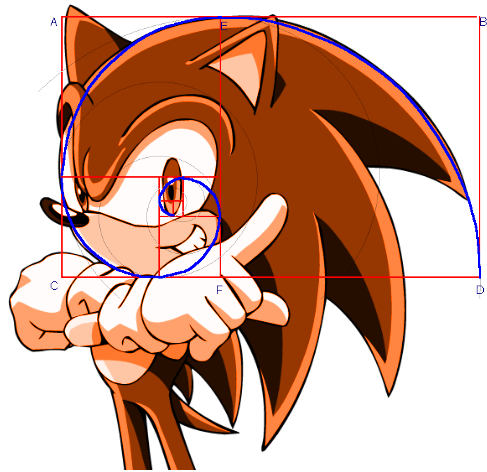## Golden number and Fibonacci sequenceFibonacci sequence is defined as follows: $$F_n = n \text{ for } n < 2$$ $$F_n = F_{n-1} + F_{n-2} \text{ otherwise}$$

1. Some sequence is defined as follows: if we take two consecutive numbers, the next number will be product of these two numbers divided by their sum. What is the $n$-th number of this sequence if first is $\frac{1}{2}$ and second is $\frac{1}{3}$?
2. How many ways can we tile a rectangular chessboard of size $2 \times n$ with dominos ($2 \times 1$)?
3. We can go upstairs by taking one step at the time or jump and take two steps. How many ways can we reach the $n$-th step?
4. How many different sequences of $n$ elements can we make from 0s and 1s if no two adjacent zeroes are allowed?
5. $n$ people are standing in the lane. We give them numbers so $i$-th person is standing on the $i$-th position. How many ways can we reaarange these poeple in such way that every person stay on the same place or on the one of adjacent ones?
6. Morse code is as sequence of finite number of dots and dashes. The length of such code is defined as the sum of weights of every symbol, where dot has weight 1 and dash - 2. Find the number of Morse codes of length $n$.
7. Prove that for every $n$: $$\sum_{i=0}^n F_i = F_{n+2} - 1$$
8. Prove that for every $n$: $$\sum_{i=0}^n F_{2i-1} = F_{2n}$$
9. Prove that for every $n$: $$F^2_{n+1} - F^2_{n-1} = F_{2n} \text{ and } F^2_{n+1} - F^2_{n} = F_{2n+1}$$
10. Prove that for every $n$: $$F_{2n} = \sum_{i-1}^n {n \choose i} F_i$$
11. Prove that for every $n$: $5|F_{5n}$.
12. Find the $F_{2015}$ modulo 10.
13. Prove that for every $n$ and $m$: $F_n | F_{mn}$.
14. Prove that for every $n$: $$F_{2n} | F_{3n} + (-1)^n F_n$$
15. Prove that for every $n > 0$ there exist a number $k$ such that $k$ is Fibonacci number and $n|k$.
16. Prove that if $F_n$ is prime, then $n$ is prime too.
17. Prove that last digits of Fibonacci numbers are periodical. Find this period.
18. Find the length of period for numbers consisting of last two digits of Fibonacci numbers.
19. Prove that in Fibonacci sequence there is a number ending with thousand of zeroes.
20. Prove that for every $n$ and $m$: $$\sum_{i=1}^n |m-i| F_i \geq F_{n+2} + F_n - n - 1$$
21. Prove that for every $n$: $$\arctan(F_{2n+1}) + \arctan(F_{2n+2}) = \arctan(F_{2n})$$
22. Prove that every natural number can be divided into finite sum of Fibonacci numbers such that no two of them are equal.
23. Prove Lucas' formula: $$F_n = \sum_{i=0} {n-i \choose i}$$
24. Prove that sums of the numbers on diagonals in Pascal triangle are Fibonacci numbers.
25. Prove that for every $n \in \mathbb{N}$: $$\sum_{i=1}^{n} \frac{1}{F_i} < 4$$

© Bartosz Kostka 2013-2018